Mein RP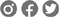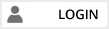Finde die besten Reiseangebote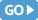Alle Veranstalter vergleichen
Fair buchen: Finde die besten Reiseangebote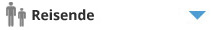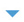1 Erwachsener
hin und zurück
oneway
Gabelflug

# Die wichtigsten IATA-Flughafen-Codes

Hier finden Sie die wichtigsten IATA-Flughafen-Codes.

Abidjan = ABJ
Abu Dhabi = AUH
Acapulco = ACA
Accra = ACC
Ajaccio = AJA
Albany = ALB
Albuquerque = ABQ
Alghero = AHO
Alicante = ALC
Alice Springs = ASP
Almeria = LEI
Amman = AMM
Amsterdam = AMS
Anchorage = ANC
Ankara = ANK
Antalya = AYT
Antananarivo = TNR
Antigua = ANU
Apia = APW
Aruba = AUA
Aspen = ASE
Astana = TSE
Asuncion = ASU
Athens = ATH
Atlanta = ATL
Auckland = AKL
Austin = AUS
Avignon = AVN
Ayers Rock = AYQ
Bahrein = BAH
Baltimore = BWI
Bamako = BKO
Bandar Seri Begawan = BWN
Bangalore = BLR
Bangkok = BKK
Banjul = BJL
Barcelona = BCN
Bari = BRI
Basel = BSL
Basel/Mulhouse = EAP
Bastia = BIA
Beijing Capital Apt = PEK
Beirut = BEY
Belem = BEL
Belfast = BFS
Belize City = BZE
Belo Horizonte = BHZ
Berlin = BER
Berlin Schonefeld Apt = SXF
Berlin Tegel Apt = TXL
Berlin Tempelhof Apt = THF
Bermuda = BDA
Biarritz = BIQ
Bilbao = BIO
Billund = BLL
Birmingham = BHX
Bogota = BOG
Bologna = BLQ
Bombay /Mumbai = BOM
Bonaire = BON
Bordeaux = BOD
Boston = BOS
Bournemouth = BOH
Bratislava = BTS
Brazzaville = BZV
Bremen = BRE
Brisbane = BNE
Bristol = BRS
Broome = BME
Brussels = BRU
Bucarest = BUH
Budapest = BUD
Buenos Aires = BUE
Cagliari = CAG
Cairns = CNS
Calcutta = CCU
Calgary = YYC
Cali = CLO
Calvi = CLY
Canberra = CBR
Cancun = CUN
Cape Town = CPT
Caracas = CCS
Cardiff = CWL
Casablanca = CAS
Cayo Coco = CCC
Cayo Largo del Sur = CYO
Cebu = CEB
Charlotte = CLT
Chiang Mai = CNX
Chiang Rai = CEI
Chicago = CHI
Chicago O'Hare International Apt = ORD
Christchurch = CHC
Ciego de Avila = AVI
Cincinnati = CVG
Cleveland = CLE
Cochabamba = CBB
Cocos Islands = CCK
Colombo = CMB
Conakry = CKY
Curacao = CUR
Dakar = DKR
Dalaman = DLM
Dallas/Fort Worth = DFW
Dar Es Salaam = DAR
Darwin = DRW
Daytona Beach = DAB
Delhi = DEL
Denpasar Bali = DPS
Denver = DEN
Des Moines = DSM
Detroit = DTT
Detroit Wayne County = DTW
Dhaka = DAC
Djerba = DJE
Doha = DOH
Dortmund = DTM
Dresden = DRS
Dubai = DXB
Dublin = DUB
Dubrovnik = DBV
Duesseldorf = DUS
Dunedin = DUD
Durban = DUR
Easter Island = IPC
Edinburgh = EDI
Edmonton = YEA
Edmonton International Apt = YEG
Entebbe/Kampala = EBB
Erfurt = ERF
Fairbanks = FAI
Faro = FAO
Florenz = FLR
Fortaleza = FOR
Frankfurt = FRA
Friedrichshafen = FDH
Ft. de France = FDF
Ft. Lauderdale = FLL
Ft. Myers SW Florida International Apt = RSW
Fuerteventura = FUE
Fukuoka = FUK
Funchal = FNC
Gaborone = GBE
Gdansk = GDN
Genf = GVA
Genua = GOA
Gerona = GRO
Glasgow = GLA
Goa = GOI
Goeteborg = GOT
Graz = GRZ
Grenoble = GNB
Guam = GUM
Guangzhou = CAN
Guatemala City = GUA
Guayaquil = GYE
Hahn Airport = HHN
Halifax, NS = YHZ
Hamburg = HAM
Hannover = HAJ
Hanoi = HAN
Harare = HRE
Havana = HAV
Helsinki = HEL
Heraklion = HER
Ho Chi Minh City = SGN
Hobart = HBA
Holguin = HOG
Hong Kong = HKG
Honolulu = HNL
Houston = HOU
Ibiza = IBZ
Innsbruck = INN
Irkutsk = IKT
Istanbul = IST
Izmir = IZM
Jakarta = JKT
Jeddah = JED
Jerez de la Frontera = XRY
Johannesburg = JNB
Kairo = CAI
Kansas City International Apt = MCI
Karachi = KHI
Karpathos = AOK
Kathmandu = KTM
Kavala = KVA
Kiev = IEV
Kilimanjaro = JRO
Kingston = KIN
Kinshasa = FIH
Klagenfurt = KLU
Kochi = COK
KoelnBonn = CGN
Koh Samui = USM
Kopenhagen = CPH
Korfu Kerkyra = CFU
Kos = KGS
Kota Kinabalu = BKI
Krabi = KBV
Kuala Lumpur = KUL
Kuching = KCH
Kuwait = KWI
La Paz = LPB
Lagos = LOS
Lahore = LHE
Lamezia Terme = SUF
Langkawi = LGK
Lanzarote = ACE
Larnaca = LCA
Las Palmas = LPA
Las Vegas = LAS
Leipzig/Halle = LEJ
Lihue, HI = LIH
Lilongwe = LLW
Lima = LIM
Linz = LNZ
Lissabon = LIS
Liverpool = LPL
Ljubljana = LJU
London = LON
London City Apt = LCY
London Gatwick Apt = LGW
London Heathrow Apt = LHR
London Luton Apt = LTN
London Stansted Apt = STN
Los Angeles = LAX
Luang Prabang = LPQ
Luxembourg = LUX
Luxor = LXR
Maastricht = MST
Mahe Island = SEZ
Malaga = AGP
Male = MLE
Malta = MLA
Managua = MGA
Manaus = MAO
Manchester = MAN
Manila = MNL
Maputo = MPM
Marrakech = RAK
Marsa Alam = RMF
Marseille = MRS
Maun = MUB
Mauritius = MRU
Medan = MES
Melbourne = MEL
Memphis = MEM
Menorca = MAH
Mexico City = MEX
Miami = MIA
Milan - Linate Apt = LIN
Milano = MIL
Milano - Malpensa Apt = MXP
Mombasa = MBA
Monastir = MIR
Montego Bay = MBJ
Montevideo = MVD
Montpellier = MPL
Montreal = YMQ
Montreal Pierre Elliott Trudeau Int Apt = YUL
Moscow = MOW
Moscow Domodedovo Apt = DME
Moscow Domodedovo Apt = DME
Moscow Sheremetyevo Apt = SVO
Mulhouse = MLH
München = MUC
MünsterOsnabrück = FMO
Muscat = MCT
Nagasaki = NGS
Nagoya = NGO
Nairobi = NBO
Nassau = NAS
Neapel = NAP
New York = NYC
New York - J F Kennedy International Apt = JFK
New York - La Guardia Apt = LGA
New York - Newark Liberty Intern. Apt = EWR
Newcastle = NCL
Nice = NCE
Noumea = NOU
Nürnberg = NUE
Ontario = ONT
Orlando = ORL
Orlando International Apt = MCO
Osaka = OSA
Osaka Kansai International Airport = KIX
Oslo = OSL
Ottawa = YOW
Palermo = PMO
Palma Mallorca = PMI
Panama City = PTY
Papeete = PPT
Paphos = PFO
Paris = PAR
Paris - Orly Apt = ORY
Paris Charles de Gaulle Apt = CDG
Peking = BJS
Penang = PEN
Perth = PER
Pescara = PSR
Phnom Penh = PNH
Phoenix = PHX
Phuket = HKT
Pisa = PSA
Pittsburgh = PIT
Pointe-a-Pitre = PTP
Porlamar = PMV
Port au Prince = PAP
Port Elizabeth = PLZ
Port Moresby = POM
Port of Spain = POS
Portland = PDX
Porto = OPO
Porto Alegre = POA
Prag = PRG
Puerto Plata = POP
Pula = PUY
Punta Cana = PUJ
Quebec = YQB
Quito = UIO
Rarotonga = RAR
Recife = REC
Reus = REU
Reykjavik = REK
Reykjavik Keflavik International Apt = KEF
Rhodes = RHO
Riga = RIX
Rijeka = RJK
Rimini = RMI
Rio de Janeiro = RIO
Rio de Janeiro International Apt = GIG
Roma = ROM
Rome Ciampino Apt = CIA
Rome Fiumicino Apt = FCO
Rostock-Laage = RLG
Rotterdam = RTM
Saarbruecken = SCN
Sal = SID
Salt Lake City = SLC
Salzburg = SZG
Samana = AZS
Samos = SMI
San Diego = SAN
San Francisco = SFO
San Jose = SJO
San Juan = SJU
San Pedro Sula = SAP
San Sebastian = EAS
Santa Cruz de la Palma = SPC
Santiago = SCL
Santiago de Compostela = SCQ
Santo Domingo = SDQ
Sao Paulo = SAO
Sao Paulo Guarulhos Intl Apt = GRU
Seattle/Tacoma = SEA
Seoul = SEL
Seoul - Incheon International Airport = ICN
Sevilla = SVQ
Shanghai = SHA
Shannon = SNN
Sharjah = SHJ
Sharm El Sheikh = SSH
Siem Reap = REP
Singapur = SIN
Split = SPU
St. Denis de la Reunion = RUN
St. Lucia = SLU
St. Maarten = SXM
St. Petersburg = LED
Stockholm = STO
Stockholm - Arlanda Apt = ARN
Strasbourg = SXB
Stuttgart = STR
Sydney = SYD
Taipei = TPE
Tallinn = TLL
Tampa/St. Petersburg = TPA
Tanger = TNG
Tehran = THR
Tel Aviv = TLV
Tenerife Sur Reina Sofia Apt = TFS
Thessaloniki = SKG
Thiruvananthapuram = TRV
Tobago = TAB
Tokio = TYO
Tokio Narita Apt = NRT
Toronto = YTO
Toronto Lester B Pearson Intl Apt = YYZ
Toulouse = TLS
Tunis = TUN
Ulaanbaatar = ULN
Valencia = VLC
Vancouver = YVR
Venezia = VCE
Victoria Falls = VFA
Vientiane = VTE
Vigo = VGO
Vilnius = VNO
Warschau = WAW
Washington = WAS
Washington Dulles International Apt = IAD
Washington Ronald Reagan National Apt = DCA
Weeze - Niederrhein Airport = NRN
Wellington = WLG
Westerland = GWT
Wien = VIE
Windhoek = WDH
Winnipeg = YWG